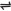## Entropy As Order-Disorder Phenomenon

The entropy of a system is a thermodynamic function, which measures the degree of randomness or disorder in the system. It is the natural tendency for any system, when left to itself, to attain the highest possible state of disorder (randomness).

Entropy is denoted as S. During change of state, systems tend to a state of greatest disorder (high entropy) as the solid changes through the liquid to the gaseous state. I.e., gaseous systems show much greater disorder than liquid systems, which in turn show greater disorder than solid systems.

Thus, systems tend to be more disordered in their final state than in their initial state. This is why it is possible to melt a lump of ice - since the molecules will assume a more disordered state in the liquid than in the solid state (ice). A state of higher disorder (liquid) is also of higher energy than the state of lower disorder (ice).

Therefore, to melt ice, heat is absorbed from the surroundings. This is also why most crystalline solids (e.g. salts) dissolve readily in water even though their dissolution in water results in a state of higher energy (since energy must be absorbed to bring about the dissolution).

When a gas is mixed with another without a change in total energy (i.e., without a reaction occurring), the molecules of the two gases which were initially separated diffuse into each other and the probability of a particular molecule of either gas occupying a given volume of space decreases during the mixing – this means that the system becomes more disordered - increase in entropy.

To Determine the Direction of Change in Entropy

1. Change of State – when a substance changes to a state of higher energy, the entropy increases, i.e. ∆S = +ve, but if the change is to a state of lower energy, entropy decreases, i.e. ∆S = -ve.

Example, H2O(l) → H2O(g) ∆S = +ve;

H2O(l) → H2O(s) ∆S = -ve.

2. Decomposition or Composition – when a substance decomposes into two or more substances, entropy increases, i.e.     ∆S = +ve, but if two or more substances compose into a single substance, entropy decreases, i.e. ∆S = -ve.

Example,

 CaCO3(s)CaO(s) + CO2(g)     ∆S = +ve
 N2(g) + 3H2(g)2NH3(g) ∆S = -ve

2HCl(s) → H2(g) + Cl2(g) ∆S = +ve

3. Change in Volume Occupied – when a gaseous substance decomposes into another gas of smaller volume, entropy decreases; but entropy increases if the product is of higher volume.

Example,

 N2O42NO2

Volume changes from 1 vol. in N2O4 to 2 vols. in NO2, therefore, entropy increases. I.e. ∆S = +ve.

Note: The entropy change involved when a system changes from an orderly state to a disorderly state is always positive         – ∆S = +ve.

From a disorderly state to an orderly state, entropy is always negative – ∆S = -ve.

Chemical reactions take place more easily at a state of high disorder (i.e. ∆S = +ve) . This is because in this state, the system is unstable (the essence of a chemical reaction is to move from states of lower stability to that of higher stability).

 Like This Post? Please Share!!!!

Copyright , All Rights Reserved Free Chemistry Online | About Us | Usage of Content | Total Disclosures | Privacy Policy